# Probability distribution of number of cycles of permutations

## Definition

Let$n$ be a natural number. Denote by$S_n$ the symmetric group of degree$n$. Consider the uniform probability measure on$S_n$ (i.e., the normalized counting measure, under which the measure of any subset is the quotient of the size of the subset by$n!$). The probability distribution of number of cycles of permutations is the probability distribution that assigns, to all numbers$1 \le k \le n$, the probability that a permutation picked uniformly at random from$S_n$ has exactly$k$ cycles in its cycle decomposition.

Note that in the count of cycles, each fixed point is treated as one cycle. Thus, the identity permutation has$n$ cycles whereas a cyclic permutation that moves all elements involves$1$ cycle.

The probability distribution assigns the following value to each$k$$\! \frac{|s(n,k)|}{n!}$

where$|s(n,k)|$ is the unsigned Stirling number of the first kind, and occurs as an important combinatorial quantity. It can also be defined as the sum, over all unordered partitions of$n$ into$k$ parts, of the sizes of the conjugacy classes corresponding to each part.

## General information

### Average information

Averaging measure Value Explanation
Mean, i.e., the expected number of cycles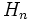$H_n$, the harmonic number of$n$, which is the sum of reciprocals of the first$n$ natural numbers Expected number of cycles of permutation equals harmonic number of degree

### Formulas in special cases

Case for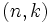$(n,k)$ Value of$|s(n,k)|$ Probability, i.e., value of$|s(n,k)|/n!$ Explanation$(n,1)$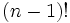$(n - 1)!$$1/n$ must be a cyclic permutation with one cycle of size$n$, then use conjugacy class size formula for symmetric group.$(n,n)$$1$$1/n!$ can only be the identity permutation.$(n,n-1)$$\binom{n}{2} = \frac{n(n-1)}{2}$$\frac{1}{2[(n-2)!]}$ must be a transposition, determined by picking a subset of size two that gets transposed.$(n,2)$,$n$ odd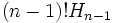$(n - 1)! H_{n-1}$, where$H_{n-1}$ is the harmonic number, i.e., the sum of reciprocals of first$n - 1$ natural numbers$H_{n-1}/n \approx (\log n)/n$

## Particular cases

The unsigned Stirling number of the first kind for any given$n$ form a unimodal sequence, hence the corresponding probability distribution is also unimodal (i.e., single-peaked).

Here is the table of unsigned Stirling numbers:$n$$n!$$|s(n,1)|$$|s(n,2)|$$|s(n,3)|$$|s(n,4)|$$|s(n,5)|$$|s(n,6)|$$|s(n,7)|$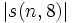$|s(n,8)|$
1 1 1
2 2 1 1
3 6 2 3 1
4 24 6 11 6 1
5 120 24 50 35 10 1
6 720 120 274 225 85 15 1
7 5040 720 1764 1624 735 175 21 1
8 40320 5040 13068 13132 6769 1960 322 28 1

Here is the table of probability values:$n$$k = 1$$k = 2$$k = 3$$k = 4$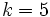$k = 5$$k = 6$$k = 7$$k = 8$
1 1
2 1/2 1/2
3 1/3 1/2 1/6
4 1/4 11/24 1/4 1/24
5 1/5 5/12 7/24 1/12 1/120
6 1/6 137/360 5/16 17/144 1/48 1/720
7 1/7 7/20 29/90 7/48 5/144 1/240 1/5040
8 1/8 363/1120 469/1440 967/5760 7/144 23/2880 1/1440 1/40320

## Computer package implementation

All unbounded variables are either defined beforehand or are replaced by actual numerical values.

Goal GAP command GAP functions used Mathematica command Mathematica functions used
Find$|s(n,k)|/n!$ Stirling1(n,k)/Factorial(n) Stirling1, Factorial Abs[StirlingS1[n,k]]/Factorial[n] Abs, StirlingS1, Factorial
List all$|s(n,k)|/n!$, fixed$n$ List([1..n],k->Stirling1(n,k)/Factorial(n)) List (Abs[StirlingS1[n, #]]/Factorial[n]) & /@ Range[n] Map, Range